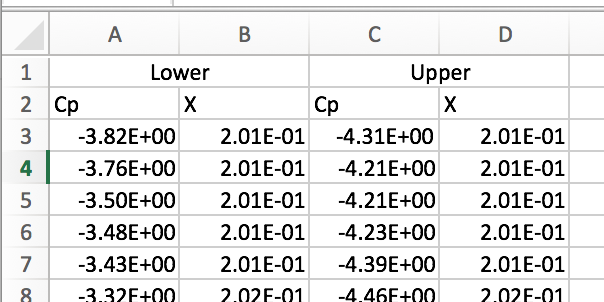# Creating a 2D Cp vs X/c graph with ParaView

I recently wanted to view the pressure distribution of a wing section and view it as a Cp vs X/c graph and remembered that this was asked about in the forums recently so I thought I would document my workflow for future references. I will start from the point where the results of the wing section or wing have been downloaded.

## Filters

ParaView has many filters so looking at the filter list and deciding the logical order in which to place them can be daunting so I shall list the filters and order they were used in, to first generate the data required.

1. Import the .case file and select only the wing surfaces for the Upper surface in the mesh regions pannel.
2. Use the ‘Extract Surfaces’ filter to create surface data from the case file.
3. Use the ‘Calculator’ filter to calculate Cp using the formula: Cp = (p - p(freestream static))/(0.5*pho(freestream)*velocity(freestream)^2)
4. Slice the surface data at the distance along the plane you wish to analyse
5. Repeat the above steps with a new instance of the .case file for the Lower Surface

The above method keeps the Upper and lower surface values separate making it easy to plot the Upper and Lower surfaces on a graph.

The resulting visualisation will look like this:

Not very fancy but holds all the data we need, finally we need to click the slices for upper and lower and save both sets of data.

## Processing the data in MS Excel (or any similar application)

Open both the .CSV files in Excel and copy and paste the Cp and X data into a new spreadsheet so you should have a layout like this:Next, create a new column and rescale the data using the formula x’ = (x-x(min))/(x(max)-x(min)) this will give all X values as values between 0 and 1 (X/c).

Finally, plot the Cp and X/c values on a graph to get the desired pressure distribution graph:

A better solution would be to plot in ParaView and save the state thus making this semi-automated, however, I have yet to grasp this.

I want to also do a write up on Cl, and Cd values of a wing in ParaView sometime in the near future.

Thanks,
Darren

3 Likes